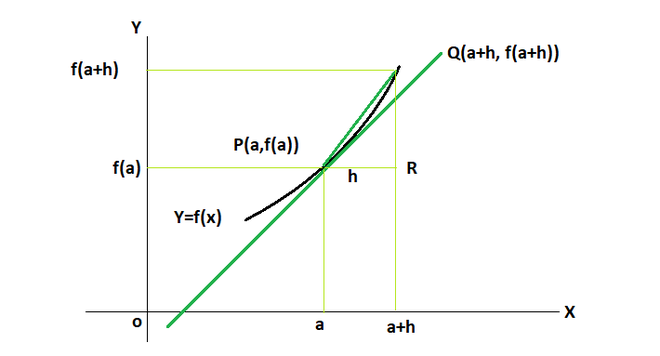GFG App
Open AppBrowser
Continue

We encounter a lot of situations in real life where we encounter some variable for which we want to know its rate of change. For example, we see a car running on the road, we are interested in its position and the rate at which its position is changing. The rate of change of position is also called velocity. In another scenario of water flowing through the water tank, we might be interested in measuring the rate at which water is flowing out of the tank. Our goal is to measure the rate at which all these variables are changing. Derivatives help in measuring the rate of the change of quantities. Let’s see how to formulate them and use them,

### Definition of Derivatives

Let’s say we have a function f(x), which we want to observe. The derivative of this function will tell us the rate at which this function is changing with respect to its input. The derivative of a function f(x) is denoted by f'(x) or. Derivatives are defined using the concept of limits.

Let’s say f is a real-valued function and ‘a’ is a point in its domain of definition. The derivative of f at a is defined as,

f'(a) =Given that it’s limits exist.

This is also referred asThe diagram given above, represents the geometric interpretation of the derivative. Lets we have two points P(a, f(a)) and Q(a + h, f(a + h)) which are close to each other on the graph. We know that according to the definition,

f'(a) =From the triangle PQR, it is clear that the ratio whose limit we are taking is precisely equal to tan(QPR) which is the slope of the chord PQ. In the limiting process, as h tends to 0, the point Q tends to P, and we have,We can see that the chord PQ tends to be a tangent to the curve f(x). The limit is equal to the slope of the tangent to the curve at the particular point.

Example: Find the derivative of f(x) = 3x at x = 2.

Solution:

f'(2) =f'(2) =⇒ f'(2) =⇒ f'(2) = 3

### Algebra of Derivatives

Since derivatives involve limits, it is expected that they should follow the properties of the limits. Let’s say we have two functions “f” and “g”.  Let’s see the properties of their derivatives.

• Derivative of the sum of two functions is equal to sum of derivatives of the two functions.• Derivatives of difference between two functions is difference of the derivatives of the two functions.• Derivatives of product of two functions is given by the product rules of the derivatives.• Derivatives of the division of the two functions is given the quotient rule of the derivatives.### Finding the equation of Tangents using derivatives

Let’s say we have a function y = f(x). We need to find the equation of tangent to this curve at x = a. We already know that the derivative at a particular point gives us the slope of the tangent. So, then we have the points (a, f(a)) and the slope of the tangent. We can form the equation of the line as given below,

Let’s say the derivative of the function at x = a,. We know that the equation of line is

y = mx + b,

where m =y =x + b

Now substituting the point (a,f(a)) in place of (x,y) in the above equation.

f(a) =a+ b

b = f(a) –a

Thus, the equation of the line becomes,

y =x + f(a) –a

Let’s see some sample problems on these concepts.

### Sample Problems

Question 1: Find the derivative of the function f(x) = x2 at x = 0.

Solution:

f'(x) =f'(x) =⇒ f'(x) =⇒ f'(x) =⇒ f'(x) = 2x

f'(0) = 0

Question 2: Find the derivative of the function f(x) = x2 at x = 2.

Solution:

f'(x) =f'(x) =⇒ f'(x) =⇒ f'(x) =⇒ f'(x) = 2x

f'(2) = 4

Question 3: Find the derivative of the function f(x) = x2 + x +1 at x = 0.

Solution:

f'(x) =f'(x) =⇒ f'(x) =⇒ f'(x) =⇒ f'(x) =⇒f'(x) = 2x + 1

f'(0) = 1

Question 4: Find the derivative of the function f(x) = ex at x = 0.

Solution:

f'(x) =f'(x) =⇒ f'(x) =⇒ f'(x) =This is 0/0 form of the limit. We know that⇒ f'(x) =⇒ f'(x) =⇒f'(x) =ex

f'(0) = 1

Notice that the derivative of exponential function is exponential itself.

Question 5: Find the equation of tangent at the point x = 0 for the given function f(x) = x2 + 1.

Solution:

We know that, f(x) = x2 + 1

f(1) = 2

So, the tangent passes through (0,2). Now to find the slope of the tangent, let’s calculate the derivative of this function.

f'(x) =f'(x) =⇒ f'(x) =⇒ f'(x) =⇒ f'(x) =⇒ f'(x) = 2x

f'(1) = 2

So, the slope of the tangent = 2.

General equation of line

y = mx + b

where m = 2, we still don’t know b

y = 2x + b

We know that this line passes through (0,2)

2 = 2(0) + b

⇒ 2 = b

Thus, equation of tangent is

y = 2x + 2

Question 6: Find the derivative of f(x) = x2 + ex

f(x) = x2 + ex

We know that this function is made up of two terms, from the previous questions we know the derivatives of these two terms individually.

So, using the properties mentioned above,We can break this function into different functions, calculate their derivatives and add them.

Let’s say f(x) = g(x) + h(x)

Where g(x) = x2 and h(x) = ex. The derivatives of these functions have been calculated already in the previous questions,

g'(x) = 2x and h'(x) = ex

f'(x) = g'(x) + h'(x)

f'(x) = 2x + ex

My Personal Notes arrow_drop_up